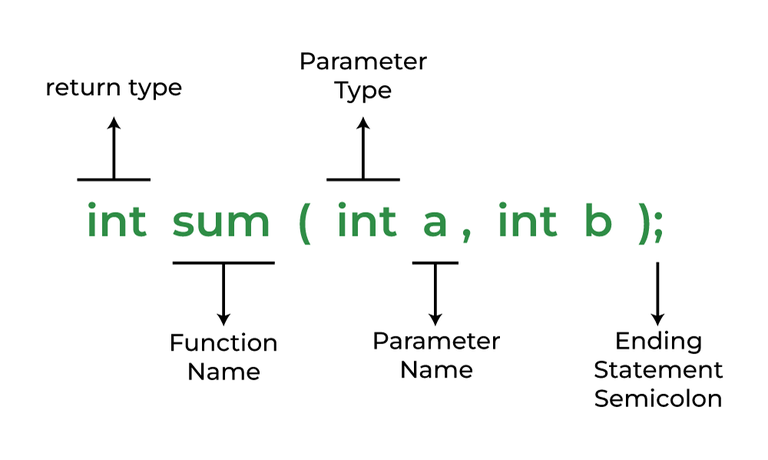Open in App
Not now

# C++ Function Call By Value

• Last Updated : 27 Jan, 2023

A function is a collection of statements that accept inputs, carry out certain calculations, and output the results. The concept is to group similar or often performed actions into a function so that we may call the function rather than writing the same code again for various inputs. A function is a section of code that executes only when it is called, to put it simply.Function in C++

### Call by Value

The call-by-value method allows you to copy the actual parameter to a formal parameter. In this case, if we change the formal parameter then the actual parameter doesn’t change. C++ uses call by value method by default.

#### Use of Call by value

Call by value is used in certain conditions like:

• When you do not want to change the actual parameters of the function.
• When you want to make copies of the data instead of the actual data.
• When space is not an issue.
• Usually, when you do not deal with recursion or backtracking.

#### Limitations of using Call by Value

Although call by value is so useful for us while programming in C++, it has a few limitations too:

• Data passed is then stored in temporary memory
• Can’t operate over the actual data rather need to work on temporary copy made of the data, because of which changes made in the data in the function is not reflected in main function.
• Memory Space required while using string ,array , vector, etc can be huge.
• Tackling Backtracking and recursion can be complex using call by values.

## C++

 `// C++ Program to implement``// Swapping using Call by function``#include ``using` `namespace` `std;` `// Swap function to demonstrate``// call by value method``void` `swap(``int` `x, ``int` `y)``{``    ``int` `t = x;``    ``x = y;``    ``y = t;``    ``cout << ``"After Swapping in function x: "` `<< x``         ``<< ``", y: "` `<< y << endl;``}` `// Driver Code``int` `main()``{``    ``int` `x = 1, y = 2;` `    ``cout << ``"Before Swapping: "``;``    ``cout << ``"x: "` `<< x << ``", y: "` `<< y << endl;` `    ``swap(x, y);` `    ``cout << ``"After Swapping: "``;``    ``cout << ``"x: "` `<< x << ``", y: "` `<< y << endl;` `    ``return` `0;``}`

Output

```Before Swapping:
x: 1, y: 2
After Swapping in function x:2, y:1
After Swapping:
x: 1, y: 2```

As, you can see that we pass two values in the swap functions x and y i.e., 1 and 2 before and after swapping them through a call-by-value method, if, we print the value of x and y then it is not changed coz the function swap create copies of x and y and swap them and their scope is inside that function only and the actual x and y values have not been modified by the swap function that why it is called call by value. If you want that the changes also reflect in the main function then you have to call it by reference and for that, you have to append the ‘&’ symbol before x and y in the formal argument.

## C++

 `// C++ Program to demonstrate``// Call by value``#include ``using` `namespace` `std;` `// Function to change value of x``int` `fun(``int` `x)``{``    ``x += 1;``    ``return` `x;``}` `// Driver Code``int` `main()``{``    ``int` `x = 3;` `    ``cout << ``"Value of X before modifying: "` `<< x << endl;` `    ``x = fun(x);``    ``cout << ``"Value of X after modifying: "` `<< x << endl;` `    ``return` `0;``}`

Output

```Value of X before modifying: 3
Value of X after modifying: 4```

Time complexity: O(1).

Space complexity: O(1).

In the above example, if you want to change the value of a variable through a function using call by value then it is only changed in that function only and the actual value of the variable where it is defined is not changed. But, if we want to use the value from the function return the value to the main function.

My Personal Notes arrow_drop_up